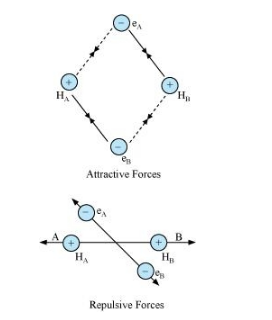# Explain the formation of H2 molecule on the basis of valence bond theory.`
Question:

Explain the formation of H2 molecule on the basis of valence bond theory.

Solution:

Let us assume that two hydrogen atoms (A and B) with nuclei (NA and NB) and electrons (eand eB) are taken to undergo a reaction to form a hydrogen molecule.

When A and B are at a large distance, there is no interaction between them. As they begin to approach each other, the attractive and repulsive forces start operating.

Attractive force arises between:

(a) Nucleus of one atom and its own electron i.e., NA – eA and NB – eB.

(b) Nucleus of one atom and electron of another atom i.e., NA – eB and NB – eA.

Repulsive force arises between:

(a) Electrons of two atoms i.e., eA – eB.

(b) Nuclei of two atoms i.e., NA – NB.

The force of attraction brings the two atoms together, whereas the force of repulsion tends to push them apart.The magnitude of the attractive forces is more than that of the repulsive forces. Hence, the two atoms approach each other. As a result, the potential energy decreases. Finally, a state is reached when the attractive forces balance the repulsive forces and the system acquires minimum energy. This leads to the formation of a dihydrogen molecule.